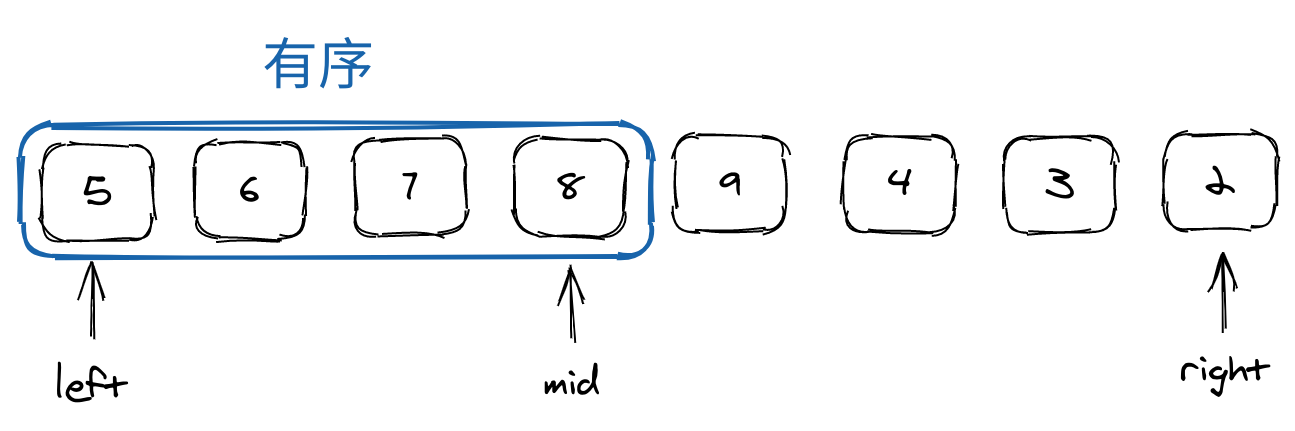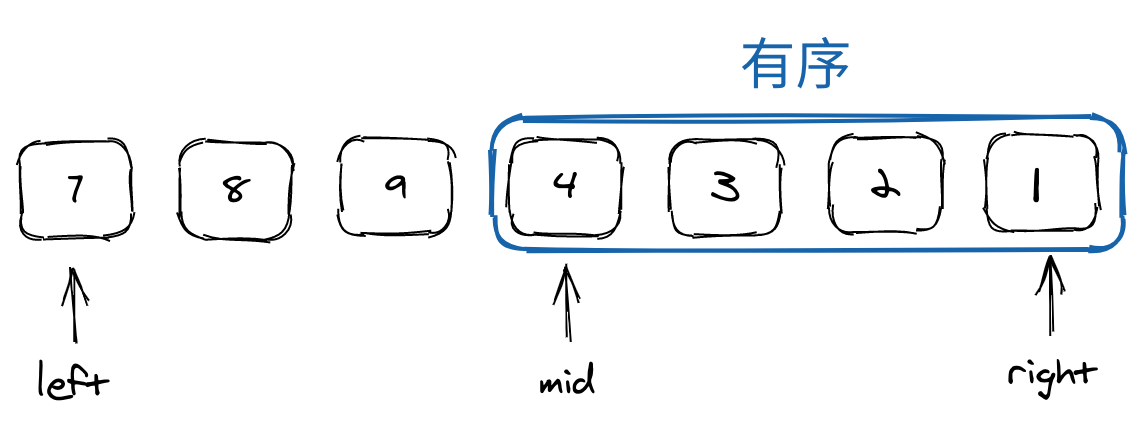# 目录

## 二分模板

• 每次都要缩减搜索区域
• 每次缩减不能排除潜在答案

### 模板 1

• 循环条件：l <= r
• 缩减搜索空间：l = mid + 1, r = mid - 1
  1 2 3 4 5 6 7 8 9 10 11  def binarySearch(nums, target): l, r = 0, len(nums)-1 while l <= r: mid = l + (r - l)//2 if nums[mid] == target: return mid # 返回索引 elif target < nums[mid]: r = mid - 1 else: l = mid + 1 return -1 

### 模板 2

• 循环条件：l < r
• 缩减搜索空间：l = mid, r = mid - 1 或者 l = mid + 1, r = mid
 1 2 3 4 5 6 7 8 9  def fuzzy_search_small(nums, target): l, r = 0, len(nums) - 1 while l < r: mid = l + (r - l + 1)//2 if nums[mid] < target: l = mid else: r = mid - 1 return nums[l] if nums[l] < target else -1 
 1 2 3 4 5 6 7 8 9  def fuzzy_search_big(nums, target): l, r = 0, len(nums) - 1 while l < r: mid = l + (r - l) // 2 if nums[mid] <= target: l = mid + 1 else: r = mid return nums[l] if nums[l] > target else -1 

### 模板 3

• 循环条件：l < r - 1
• 缩减搜索空间：l = mid, r = mid
  1 2 3 4 5 6 7 8 9 10 11 12 13 14 15 16 17 18  def fuzzy_search(nums, target): l, r = 0, len(nums)-1 while l < r - 1: mid = l + (r-l)//2 if nums[mid] == target: return [mid] # 返回索引 elif nums[mid] < target: l = mid else: r = mid tmp = nums[l]/2.0 + nums[r]/2.0 # 中间值 if target == tmp: return [l, r] elif target < tmp: return [l] else: return [r] 

## 每日一题

### 69. x 的平方根

https://leetcode-cn.com/problems/sqrtx/

Note

#### 思路

• 解 $\sqrt{n} \leq \frac{n}{2}$:
• $n = 0$, return 0
• $1 \leq n \leq 3$, return 1
• $n \geq 4$, 满足 $\sqrt{n} \leq \frac{n}{2}$
• 在 $\left[1, n//2\right]$ 内二分
• 利于除法判断: mid > x/mid, 避免溢出

#### 代码

Python
  1 2 3 4 5 6 7 8 9 10 11  class Solution: def mySqrt(self, x: int) -> int: if x == 0: return 0 i, j = 1, x // 2 while i < j: mid = i + (j-i+1) // 2 if mid > x/mid: j = mid - 1 else: i = mid return i 

#### 复杂度

• 时间复杂度：$O(logX)$
• 空间复杂度：$O(1)$

### 278. 第一个错误的版本

#### 题目描述

Note

给定 n = 5，并且 version = 4 是第一个错误的版本。



#### 思路

• 简单二分思路
• 相当于二元递增数组 [0, 0, 0, 1, 1]
• 找到第一个 1

#### 代码

Python
  1 2 3 4 5 6 7 8 9 10 11 12 13 14 15 16 17 18 19  # The isBadVersion API is already defined for you. # @param version, an integer # @return an integer # def isBadVersion(version): class Solution: def firstBadVersion(self, n): """ :type n: int :rtype: int """ i, j = 1, n while i < j: mid = i + (j-i) // 2 if isBadVersion(mid): j = mid else: i = mid + 1 return i 

#### 复杂度

• 时间复杂度：$O(logN)$
• 空间复杂度：$O(1)$

### Triple Inversion

https://binarysearch.com/problems/Triple-Inversion

#### 题目描述

Note

Given a list of integers nums, return the number of pairs i < j such that nums[i] > nums[j] * 3.

Constraints

• n ≤ 100,000 where n is the length of nums

Example

• Input
nums = [7, 1, 2]
• Output
2
• Explanation
We have the pairs (7, 1) and (7, 2)

#### 思路

• 构造递增数组 d
• 借助 bisect 模块，边插入边排序

#### 代码

Python
 1 2 3 4 5 6 7 8  class Solution: def solve(self, nums): res, d = 0, [] for num in nums: idx = bisect.bisect_right(d, 3*num) # 返回最右侧索引 res += len(d) - idx bisect.insort(d, num) return res 

#### 复杂度

• 时间复杂度：$O(n^2)$
• 空间复杂度：$O(n)$

Note

#### 代码

Python
 1   

• 时间复杂度：
• 空间复杂度：

## 推荐题目

### 33. 搜索旋转排序数组

https://leetcode-cn.com/problems/search-in-rotated-sorted-array/

#### 题目描述

Note

• $1 \leq nums.length \leq 5000$
• $-10^4 \leq nums[i] \leq 10^4$
• nums 中的每个值都 独一无二
• nums 肯定会在某个点上旋转
• $-10^4 \leq target \leq 10^4$

#### 思路

• 二分法，每次必须缩小区间，不能去掉可能解
• nums[left] <= nums[mid] $\Longrightarrow$ 左侧为有序序列• nums[left] > nums[mid] $\Longrightarrow$ 右侧为有序序列#### 代码

Python
  1 2 3 4 5 6 7 8 9 10 11 12 13 14 15 16 17  class Solution: def search(self, nums: List[int], target: int) -> int: left, right = 0, len(nums)-1 while left <= right: mid = left + (right-left)//2 if nums[mid] == target: return mid if nums[left] <= nums[mid]: # 左侧为有序序列 if nums[left] <= target < nums[mid]: right = mid-1 else: left = mid+1 else: # 右侧为有序序列 if nums[mid] < target <= nums[right]: left = mid+1 else: right = mid-1 return -1 

#### 复杂度

• 时间复杂度：$O(logN)$
• 空间复杂度：$O(1)$

### 81. 搜索旋转排序数组 II

https://leetcode-cn.com/problems/search-in-rotated-sorted-array-ii/

#### 题目描述

Note

( 例如，数组 $[0,0,1,2,2,5,6]$ 可能变为 $[2,5,6,0,0,1,2]$ )。

#### 思路

 1 2  while left < mid and nums[left] == nums[mid]: # 消除重复元素 left += 1 

#### 代码

Python
  1 2 3 4 5 6 7 8 9 10 11 12 13 14 15 16 17 18 19  class Solution: def search(self, nums: List[int], target: int) -> bool: left, right = 0, len(nums)-1 while left <= right: mid = left + (right-left)//2 if nums[mid] == target: return True while left < mid and nums[left] == nums[mid]: left += 1 # 消除重复元素 if nums[left] <= nums[mid]: # 左侧为有序序列 if nums[left] <= target < nums[mid]: right = mid-1 else: left = mid+1 else: # 右侧为有序序列 if nums[mid] < target <= nums[right]: left = mid+1 else: right = mid-1 return False 

#### 复杂度

• 时间复杂度：$O(logN)$
• 空间复杂度：$O(1)$